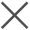• 机器数据分析平台 > 使用文档 > 应用平台 > Pandora机器学习工具包 >fit的使用方法详述 > 预测离散指标【分类】 > 多层感知机分类器 - MLPClassifier

# 多层感知机分类器 - MLPClassifier

最近更新时间: 2022-02-24 16:19:05

多层感知机是一种简单的多层神经网络模型。可以适用于二分类或者多分类场景。

``````..| fit MLPClassifier batch_size=<int> max_iter=<int> random_state=<int> tol=<floats> momentum=<floats> learning_rate_init=<float> power_t=<float> activation=<identity | logistic | tanh | relu(default)> solver=<lbfgs | sgd | adam(default)> learning_rate=<constant(constant) | invscaling | adaptive> hidden_layer_sizes=<tuple> <target_field> from <feature_field_1> <feature_field_2>...
``````

参数说明：

• batch参数用来指定mini-batch sgd优化器的样本批量大小，默认值为200(如样本数低于200，则为样本数)。

• max_iter用来指定神经网络的最大迭代次数，默认值为200。

• random_state用来指定随机种子，用来控制模型初始权重的随机性。如果给定特定值，重新跑模型的时候，可以得出同样的结果。

• tol参数用于指定优化器的忍耐度。当损失函数的值的变化小于了忍耐度，便认为训练结束，从而停止训练。默认值为1e-4。

• momentum参数用于指定随机梯度下降优化器的动量，其值介于0到1之间。该参数仅在优化器为sgd的情形下有效。

• activation用来指定激活函数。

• activation = identity，使用f(x) = x的激活函数
• activation = logistic，使用f(x) = 1 / (1 + exp(-x))的激活函数，又名sigmoid激活函数。
• activation = tanh，使用双曲正切激活函数。
• activation = relu，使用整流线性单元激活函数。（默认值）
• solver用来指定优化器。

• solver = lbfgs,使用拟牛顿法作为优化器。
• solver = sgd,使用随机梯度下降优化器。
• learning_rate参数用来指定学习率的模式。

• learning_rate = constant，即使用常数作为学习率。用learning_rate_init参数来设置其值。(默认值)
• learning_rate = invscaling，使用一种逐渐自动下降的学习率。在训练的第t步，学习率会变为learning_rate_init / pow(t, power_t)。其中power_t可以手动设定，其默认值为0.5。
•